# Risk Assessment of Smart Community Heating Reconstruction Project Based on Artificial Neural Network

Risk Assessment of Smart Community Heating Reconstruction Project Based on Artificial Neural Network
School of Mathematics and Science, Nanyang Institute of Technology, Nanyang 473004, China

Corresponding Author Email:
jfqiao@nyist.edu.cn
Page:
867-875
|
DOI:
https://doi.org/10.18280/ijht.390321
3 February 2021
|
Accepted:
9 May 2021
|
Published:
30 June 2021
| Citation

OPEN ACCESS

Abstract:

With the rapid development of urbanization, energy conservation has become an important measure for building a conservation-oriented harmonious society. The smart reconstruction of the heating system in old communities is now an inevitable choice for urban development. However, there is not yet any unified risk assessment system for these smart heating reconstruction projects. By virtue of the advantages of artificial neural network (ANN) in data processing, this paper tries to assess the risks of smart community heating reconstruction projects. Firstly, a risk assessment system was established for smart community heating reconstruction projects, and the sensitivity of the indices was analyzed. Next, the primary and secondary models for risk assessment were constructed, and the reliability of project investment risk assessment was examined. Finally, artificial fish swarm algorithm (AFSA) was adopted to optimize the initial connection weights and thresholds of backpropagation neural network (BPNN), and the AFSA-optimized network was adopted to build a risk assessment model for smart community heating reconstruction projects. The feasibility and effectiveness of the proposed model were verified through experiments.

Keywords:

neural network, heating reconstruction, risk assessment, artificial fish swarm algorithm (AFSA)

1. Introduction

With the rapid development of urbanization, there is a growing requirement on the comprehensive utilization of resources and energy, as well as environmental protection. Energy conservation has become an important measure for building a conservation-oriented harmonious society [1-4]. Considering the low per-capita quantity of resources and energy in China, the source control of energy waste is of great significance to national progress and development [5-8]. Therefore, the smart reconstruction of the heating system in old communities is now an inevitable choice for urban development [9-11]. But there are risks in any reconstruction project. To measure the values of construction and investment, it is necessary to develop a perfect risk assessment system.

Based on the definition of the concepts in the risk management of heating reconstruction, Wu et al.  detailed six risk identification methods, including Delphi and brainstorming, examined the features of the original heating system of communities, and designed a relatively complete risk evaluation system for heating reconstruction. Aiming to reduce the energy consumption of centralized heating to high-pollution, high-energy industrial zones, Huang et al.  relied on the relevant theory to elaborate on the principles of techno-economic appraisal of the centralized heating reconstruction of industrial zones, analyzed the risks and sustainability of the project, and evaluated the macroeconomic impacts of the region.

Heating reconstruction faces multiple risks in terms of environment, technology, and management. Any accident would bring grave losses [14-19]. Kim and Kwon  discussed the universal problems in the quality management of heating reconstruction, proposed a quality management model led by the quality control (QC) team, and recommended to improve the quality assurance (QA) system for the key processes before, during, and after construction. Starting from the features of old community heating system, Kawasaki et al.  summarized and analyzed the features, components, and technical means, examined an example of heating reconstruction in a hot summer cold winter region, and predicted the development model and direction of centralized heating in the region, using strength, weakness, opportunity, and threat (SWOT) analysis and entropy weight method.

At present, it is an inevitable trend to promote the smart reconstruction of heating in old communities. But these projects have complex technologies and high risks [22, 23]. However, there is not yet any unified risk assessment system for these smart heating reconstruction projects. The lack of the system slows down project execution, and adds to the difficulty of risk control [24-27]. By virtue of the advantages of artificial neural network (ANN) in data processing, this paper tries to assess the risks of smart community heating reconstruction projects. The main contents of this work are as follows: (1) Building a risk assessment system for smart community heating reconstruction projects, and analyzing the sensitivity of the indices; (2) Setting up the primary and secondary models for risk assessment, and examining the reliability of project investment risk assessment; (3) Adopting artificial fish swarm algorithm (AFSA) to optimize the initial connection weights and thresholds of backpropagation neural network (BPNN), and establishing a risk assessment model for smart community heating reconstruction projects based on the AFSA-optimized BPNN. Experimental results demonstrate the feasibility and effectiveness of the proposed model.

2. Risk Assessment System

Smart community heating reconstruction has just started in China. There are not many technologies or investment cases for reference. Besides, any community heating reconstruction project faces lots of uncertainties, which arise from the geographic location, layout, and building features of the communities. Similarly, uncertain factors abound throughout project construction. To a certain extent, the distribution law and change mechanism of these factors are stochastic and fuzzy. The stochasticity and fuzziness must be fully considered to assess the risks of smart community heating reconstruction projects.

Figure 1 shows the risk assessment model for smart community heating reconstruction projects. To improve the accuracy of project decision-making, this paper firstly constructs a fuzzy set that is randomly projected by the law of large numbers, and then evaluates the smart community heating reconstruction risks based on fuzzy mathematics and set-valued statistics.

## 1.png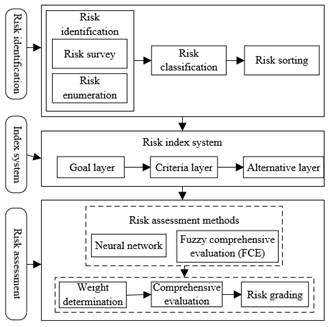Figure 1. Risk assessment system for smart community heating reconstruction projects

This paper constructs a hierarchical risk assessment system for smart community heating reconstruction projects, which covers four aspects: market aspect, technical design, project investment, and heating reliability.

Layer 1 (goal layer):

HRR={risk assessment of smart community heating reconstruction};

Layer 2 (criteria layer):

HRR={HRR1, HRR2, HRR3, HRR4}={market aspect, technical design, project investment, heating reliability};

Layer 3 (alternative layer):

HRR1={HRR11, HRR12, HRR13, HRR14, HRR15, HRR16}={real-time electricity price, real-time heating price, heat source heating cost, grid power supply cost, risk of public opinion, operating risk of reconstructed system};

HRR2={HRR21, HRR22, HRR23, HRR24, HRR25, HRR26, HRR27, HRR28, HRR29}={rationality of technical solution, completeness of equipment, professionalism of personnel, reliability of installation and commissioning, controllability of project construction and coordination, operating stability of original and reconstructed system, reliability of power supply, safety of relay protection, protection of power quality};

HRR3={HRR31, HRR32, HRR33, HRR34, HRR35, HRR36, HRR37, HRR38}={investment fund, construction cycle, operating investment cost, additional investment cost, energy efficiency evaluation, profitability, development capacity, operating capacity};

HRR4={HRR41, HRR42, HRR43, HRR44, HRR45, HRR46}={operating reliability, equipment aging, equipment damage, pipeline aging, climate and geographical factors, generator power}.

Out of the 28 secondary indices, the indices that significantly affect the risk assessment of smart community heating reconstruction and change greatly over time were taken as risk factors. Let vi be the i-th risk factor. Then, the set of risk factors can be expressed as:

$V=\left\{ {{v}_{1}},{{v}_{2}},...,{{v}_{m}} \right\}$      (1)

This paper defines the degree of variation of a risk assessment index with influencing factors as the sensitivity of its risk factor. The sensitivity ξi of an index to a risk factor vi can be expressed as:

${{\xi }_{i}}=\left| \frac{\frac{dHHR}{HHR}}{\frac{d{{v}_{i}}}{{{v}_{i}}}} \right|=\left| \frac{dHHR}{d{{v}_{i}}}\frac{{{v}_{i}}}{HHR} \right|\text{ }\left( i=1,2,...,n \right)$       (2)

Similarly, the sensitivity ξi(l) of index HHR to the i-th risk factor vi in period l can be expressed as:

$\xi _{_{i}}^{\left( l \right)}=\left| \frac{\frac{dHH{{R}^{\left( l \right)}}}{HH{{R}^{\left( l \right)}}}}{\frac{dv_{_{i}}^{\left( l \right)}}{v_{_{i}}^{\left( l \right)}}} \right|=\left| \frac{dHH{{R}^{\left( l \right)}}}{dv_{_{i}}^{\left( l \right)}}\frac{v_{_{i}}^{\left( l \right)}}{HH{{R}^{\left( l \right)}}} \right|\text{ }\left( i=1,2,...,n;l=1,2,...,m \right)$       (3)

The value of ξi can be calculated by:

${{\xi }_{i}}=\frac{1}{m}\sum\limits_{l=1}^{m}{\xi _{_{i}}^{\left( l \right)}}\text{ }\left( i=1,2,...,n \right)$       (4)

The normalized value ξi* of ξi can serve as the weight coefficient of risk factors:

$\xi _{i}^{*}=\frac{{{\xi }_{i}}}{\sum\limits_{i=1}^{n}{{{\xi }_{i}}}}\text{ }\left( i=1,2,...,n \right)$      (5)

Table 1 lists the sensitivities of the four primary indices.

Table 1. Sensitives of primary indices

 Primary indices -15% -10% -5% 0% 5% 10% 15% Market aspect 16.35 23.62 26.79 28.64 31.79 35.65 42.03 Technical design 16.35 23.62 26.79 28.64 31.79 35.65 42.03 Project investment 18.55 24.34 27.18 28.64 32.08 33.64 34.51 Heating reliability 31.62 30.71 30.51 28.64 28.86 29.14 28.54.
3. Pimary Model for Risk Assessment

## 2.pngFigure 2. Risk assessment flow

Following the risk assessment flow in Figure 2, this paper constructs the membership function for the risk assessment through statistical method. Firstly, a fuzzy set and a fuzzy matrix were established for the risk assessment. Let U={u1, u2, …, u5} be the set of judged risk grades, i.e., the grades of risk factors, corresponding to the set of risk factors V={v1, v2, …, vm}. Then, the curve of the membership function for the fuzzy set of risk factors was plotted based on fuzzy statistics, and used to derive the membership function:

{{\lambda }_{i1}}=\left\{ \begin{align} & 1\text{ }{{e}_{i0}}\le a\le {{e}_{i1}} \\ & \frac{{{e}_{i2}}-a}{{{e}_{i2}}-{{e}_{i1}}}\text{ }{{e}_{i1}}\le a\le {{e}_{i2}} \\ & 0\text{ }{{e}_{i2}}\le a\le {{e}_{in}} \\\end{align} \right.\text{ }\left( i=1,2,...,n \right)      (6)

{{\lambda }_{ij}}=\left\{ \begin{align} & 0\text{ }{{e}_{i0}}\le a\le {{e}_{i,j-2}} \\ & \frac{a-{{e}_{i,j-2}}}{{{e}_{i,j-1}}-{{e}_{i,j-2}}}\text{ }{{e}_{i,j-2}}\le a\le {{e}_{i,j-1}} \\ & 1\text{ }{{e}_{i,j-1}}\le a\le {{e}_{ij}} \\ & \frac{{{e}_{i,j+1}}-a}{{{e}_{i,j+1}}-{{e}_{ij}}}\text{ }{{e}_{ij}}\le a\le {{e}_{i,j+1}} \\ & 0\text{ }{{e}_{i,j+1}}\le a\le {{e}_{in}} \\\end{align} \right.\text{ }\left( i=1,2,...,n,j=2,...,n-1 \right)       (7)

{{\lambda }_{in}}=\left\{ \begin{align} & 0\text{ }{{e}_{i0}}\le a\le {{e}_{i,m-2}} \\ & \frac{a-{{e}_{i,m-2}}}{{{e}_{i,m-1}}-{{e}_{i,m-2}}}\text{ }{{e}_{i,m-2}}\le a\le {{e}_{i,m-1}} \\ & 1\text{ }{{e}_{i,m-1}}\le a\le {{e}_{in}} \\\end{align} \right.\text{ }\left( i=1,2,...,n \right)       (8)

Let [alki, alhi] be the value of the given risk factor vi estimated by each investor al in smart community heating reconstruction in period l. Then, we have:

$a_{i}^{l}=\frac{{{a}^{lk}}+a_{i}^{lh}}{2}$        (9)

By formula (9), a set of risk estimates bl=(a1, a2, …, aln) could be obtained, where bl is the value of risk factor vi estimated by investor al.

Let vij(bl) be the degree of al belonging to uj relative to vi. Then, the obtained ali value can be substituted to the membership function to obtain the value of vij(bl). Then, the fuzzy evaluation matrix Ŝl between v and u of al can be established as:

${{\hat{S}}^{l}}=\left( \begin{matrix} {{v}_{11}}\left( {{b}^{l}} \right) & {{v}_{12}}\left( {{b}^{l}} \right) & \cdots & {{v}_{1m}}\left( {{b}^{l}} \right) \\ {{v}_{21}}\left( {{y}^{k}} \right) & {{v}_{22}}\left( {{b}^{l}} \right) & \cdots & {{v}_{2m}}\left( {{b}^{l}} \right) \\ \vdots & \vdots & \vdots & \vdots \\ {{v}_{n1}}\left( {{b}^{l}} \right) & {{v}_{n2}}\left( {{b}^{l}} \right) & \cdots & {{v}_{nn}}\left( {{b}^{l}} \right) \\\end{matrix} \right)$      (10)

Let sij=vij(bl) be the membership of vi relative to uj. To compare the memberships between risk factors, each row of Ŝl can be normalized by:

$s_{ij}^{*}=\frac{{{v}_{ij}}}{{{v}_{i1}}+{{v}_{i2}}+...+{{v}_{in}}}$     (11)

Based on the fuzzy evaluation matrix and risk factor sensitivities, the following primary risk assessment model can be established:

${{\hat{F}}^{l}}={{\xi }^{*}}\circ {{\hat{S}}^{l}}=\left( {{f}_{1}},{{f}_{2}},...,{{f}_{m}} \right)$      (12)

where, fj can be calculated by:

${{f}_{j}}=\sum\limits_{i=1}^{n}{\xi _{i}^{*}s_{ij}^{*}}\left( j=1,2,...,m \right)$      (13)

4. Reliability Analysis

Lacking investment experience, the decision-makers want to make objective decisions about whether or not to invest in smart community heating reconstruction through quantitative analysis of the reconstruction project. If expert method is adopted to evaluate the risk attributes of the project, the evaluation will be greatly affected by the expertise and preference of the experts. The set-valued statistics provides an effective way to ease the subjective interference. This approach views a fuzzy risk factor as a random variable that can be characterized with an interval. By set-valued statistics, the risk factors can be counted in a fuzzy manner, while making objective decisions and fuzzy judgements. The interval of the value of a risk factor estimated by the i-th expert can be defined as:

${{\sigma }_{i}}=\left[ {{a}_{i,}}a_{i}^{'} \right]\text{ }\left( j=1,2,...,k \right)$      (14)

According to the principle of set-valued statistics, the assessment index of the risk factor can be described by a convex membership function Ã:

$\tilde{A}=g\left( a \right)=\frac{1}{k}\left( \sum\limits_{i=1}^{k}{\Phi _{{{\sigma }_{i}}}^{\left( a \right)}} \right)$      (15)

where, Φσi(a) is a binary function reflecting whether a falls in the interval of estimated value:

\Phi _{_{{{\sigma }_{i}}}}^{\left( a \right)}=\left\{ \begin{align} & 1\text{ }a\in \left[ {{a}_{i,}}a_{j}^{'} \right]={{\delta }_{i}} \\ & 0\text{ }a\notin \left[ {{a}_{i,}}a_{j}^{'} \right]={{\delta }_{i}} \\\end{align} \right.     (16)

Combining formulas (15) and (16):

\tilde{A}=g\left( a \right)=\left\{ \begin{align} & {{l}_{1}}\text{ }a\in \left[ {{e}_{1}},{{e}_{2}} \right) \\ & {{l}_{2}}\text{ }a\in \left[ {{e}_{2}},{{e}_{3}} \right) \\ & \vdots \text{ }\vdots \\ & {{l}_{m}}\text{ }a\in \left[ {{e}_{m}},{{e}_{m+1}} \right] \\\end{align} \right.      (17)

Ã can be normalized as:

$\int_{{{e}_{1}}}^{{{e}_{n+1}}}{\overset{\tilde{\ }}{\mathop{A}}\,{{r}_{a}}}=\sum\limits_{i=1}^{m}{{{l}_{i}}}\left( {{e}_{i+1}}-{{e}_{i}} \right)=p$      (18)

Then, the normalized convex membership function of Ã can be expressed as:

\dot{A}=\dot{g}\left( x \right)=\left\{ \begin{align} & \frac{{{l}_{1}}}{p}\text{ }a\in \left[ {{e}_{1}},{{e}_{2}} \right) \\ & \frac{{{l}_{2}}}{p}\text{ }a\in \left[ {{e}_{1}},{{e}_{2}} \right) \\ & \vdots \text{ }\vdots \\ & \frac{{{l}_{m}}}{p}\text{ }a\in \left[ {{e}_{m}},{{e}_{m+1}} \right] \\\end{align} \right.     (19)

The normalized convex membership function is constrained by:

$\int_{{{e}_{1}}}^{{{e}_{n+1}}}{\dot{A}{{r}_{a}}}=1$      (20)

The expectation DV(A) of the assessment index of the risk factor can be given by:

$DV\left( A \right)=\int_{{{e}_{1}}}^{{{e}_{m+1}}}{\overset{\hat{\ }}{\mathop{A}}\,ada}=\frac{1}{2p}\sum\limits_{i=1}^{m}{{{l}_{i}}}=\left( e_{_{i+1}}^{2}-e_{_{i}}^{2} \right)$      (21)

The corresponding variance VC(A) can be described by:

\begin{align} & VC\left( A \right)=\int_{{{e}_{1}}}^{{{e}_{m+1}}}{\overset{\hat{\ }}{\mathop{A}}\,{{\left[ a-DV\left( A \right) \right]}^{2}}r\left( a \right)}= \\ & \frac{1}{3p}\sum\limits_{i=1}^{m}{{{l}_{i}}}\left\{ {{\left[ e_{_{i+1}}^{2}-DV\left( A \right) \right]}^{3}}-{{\left[ {{e}_{i}}-DV\left( A \right) \right]}^{3}} \right\} \\\end{align}      (22)

The standard deviation BD(A) can be calculated by:

$BD\left( A \right)=\sqrt{VC\left( A \right)}$      (23)

Then, the interval of the maximum possible value of the assessment index for the risk factor can be defined as:

$VC=\left[ DV\left( A \right)-BD\left( A \right),DV\left( A \right)+BD\left( A \right) \right]$      (24)

Suppose the interval satisfies:

${{\sigma }_{i}}\cap VC=\left[ {{r}_{i}},r_{_{i}}^{'} \right]\text{ }\left( i=1,2,...,k \right)$     (25)

The expected estimation fuzziness wij of the i-th expert for the j-th risk factor can be calculated by:

${{w}_{ij}}=r_{_{i}}^{'}-{{r}_{i}}$     (26)

The expected estimation fuzziness w'j of all experts for the j-th risk factor can be calculated by:

$w_{j}^{'}=\sum\limits_{i=1}^{k}{{{w}_{ij}}}$      (27)

If the experts are uncertain about the estimated interval, wij=0. The assessment reliability ηij of the i-th expert for the j-th risk factor can be calculated by:

${{\eta }_{ij}}=\frac{{{w}_{ij}}}{2BD\left( A \right)}\text{ }\left( i=1,2,...,k \right)$      (28)

The comprehensive assessment reliability S of all experts for the j-th risk factor can be calculated by:

$S=\frac{w_{j}^{'}}{2BD\left( A \right)}$      (29)

The normalized result θij of ηij can be expressed as:

${{\theta }_{ij}}=\frac{{{s}_{ij}}}{\sum\limits_{i=1}^{k}{{{s}_{ij}}}}$      (30)

θij satisfies Σki=1θij=1. Formula (30) shows that the θij value increases with the level of the i-th expert. Smart community heating reconstruction involves many risk factors. Therefore, the assessment of the reconstruction risks is a multi-factor comprehensive assessment. If multiple experts are invited to assess the risks of the heating reconstruction in the same smart community, then the assessment reliability Wi of the i-th expert for all risk factors can be quantified by:

${{W}_{i}}=\frac{1}{n}\sum\limits_{j=1}^{n}{{{\theta }_{ij}}}\text{ }\left( i=1,2,...,k \right)$      (31)

Wi characterizes the reliability of the subjective, empirical judgement of each expert. It can be taken as the weight coefficients of secondary indices. Since W={W1, W2, …, Wk} satisfies Σki=1Wi=1, the risk assessment model for smart community heating reconstruction can be given by:

$S=W\cdot F=\left( {{f}_{1}},{{f}_{2}},...,{{f}_{m}} \right)$      (32)

where, fj can be calculated by:

${{f}_{j}}=\sum\limits_{i=1}^{k}{{{W}_{i}}}{{f}_{ij}}\left( j=1,2,...,m \right)$      (33)

By the principle of maximum membership, fj= max{f1, f2, …, fm}. The risk grade of the target smart community heating reconstruction project is denoted as grade uj.

5. Risk Assessment Model Based on Improved BPNN

Traditional BPNN has several limitations: too many parameters are involved in training; the network is prone to falling in the local optimum trap; the samples must be selected very carefully. To overcome these limitations, this paper optimizes the initial connection weights and thresholds of the network by AFSA. Based on the AFSA-optimized BPNN, a risk assessment model was constructed for smart community heating reconstruction. The optimization process is explained in Figure 3.

## 3.png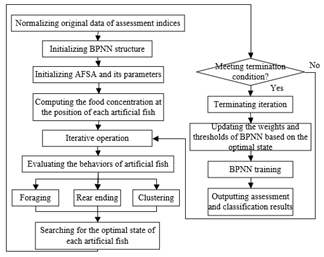Figure 3. Flow of AFSA optimization of BPNN

Artificial fishes have three basic behaviors: foraging, clustering, and rear ending. Besides, each artificial fish can perceive the environmental changes (e.g., food concentration in the surroundings, and the positions of other fishes) in real time, and determine the moving direction in the next step based on the perceived changes.

The foraging behavior is defined as the spontaneous swimming in the direction of high food concentration. Let Lτi be the current location of an artificial fish at time τ; Lj be a new location randomly selected in the view SY of the fish. Then, we have:

\left\{ \begin{align} & {{L}_{j}}=L_{i}^{\tau }+SY*Rand\left( {} \right) \\ & DI{{S}_{ij}}=\left\| L_{i}^{\tau }-{{L}_{j}} \right\|<SY \\\end{align} \right.     (34)

Let Lτ+1i be the target location of the artificial fish at Lτi in the next step. During the optimization process, if the food concentration ci at the current location Lτi is lower than that cj at the new location, then the artificial fish will swim in the direction of high food concentration cj:

$L_{i}^{\tau +1}=L_{i}^{\tau }+\frac{{{L}_{j}}-L_{i}^{\tau }}{\left\| {{L}_{j}}-L_{i}^{\tau } \right\|}$     (35)

If the food concentration ci at the current location Lτi is higher than that cj at the new location, then the artificial fish will select a new location in its view, and judge whether the food concentration cj at the new location is lower than ci, in order to determine the moving direction in the next step. If the target location is not found within the preset number of attempts, the artificial fish at Lτi will swim in a random direction in its view SY:

$L_{i}^{\tau +1}=L_{i}^{\tau }+BC*Rand\left( {} \right)$      (36)

The clustering behavior is defined as the spontaneous swimming of artificial fishes in the same direction. Before simulating this behavior, the clustering rules should be configured to prevent the fishes from overcrowding. Suppose there are mF artificial fishes in the view of the artificial fish at location Lτi. Let γ be the current crowding degree of the fish swarm; cCL be the food concentration at the center CL of mF adjacent fishes. If CL has sufficient food and a low crowding degree, then cCL/mF>γ*ci. In this case, the artificial fishes at Lτi will swim in the direction of CL:

$L_{i}^{\tau +1}=L_{i}^{\tau }+\frac{{{L}_{Z}}-L_{i}^{\tau }}{\left\| {{L}_{Z}}-L_{i}^{\tau } \right\|}*BC*Rand\left( {} \right)$     (37)

If formula (37) is not satisfied, then the artificial fishes at Lτi will swim by the foraging rules in formulas (35) and (36).

The rear ending behavior is defined as an artificial fish swimming to another artificial fish at the location with the maximum food concentration in its view. Suppose the artificial fish at Lτi looks for the adjacent fish in the location Lmax with the highest food concentration cFC-max in its view SY. If Lmax has sufficient food and a low crowding degree, then cFC-max/mF>γ* ci. Thus, we have:

$L_{i}^{\tau +1}=L_{i}^{\tau }+\frac{{{L}_{max}}-L_{i}^{\tau }}{\left\| {{L}_{max}}-L_{i}^{\tau } \right\|}*BC*Rand\left( {} \right)$      (38)

If formula (38) is not satisfied, then the artificial fishes at Lτi will swim by the foraging rules in formulas (35) and (36).

6. Experiments and Results Analysis

This section firstly analyzes the internal correlations between the estimated intervals of the risk assessment indices for smart community heating reconstruction. Table 2 sorts the secondary indices by the cumulative variance explained (CVE).

Table 2 shows that the CVE of secondary indices contained eight eigenvalues that are greater than 1: 4.427, 4.128, 2.315, 2.159, 1.721, 1.434, 1.190, and 1.021. The CVE of the eight secondary indices reached 82.489%, suggesting that these indices can reflect most risk features of Chinese old community heating reconstruction projects. Similarly, eight key risk factors could be extracted from the scree plot of the risk assessment system (Figure 4).

Table 2. CVE of each risk assessment index

 Index Eigenvalue Index Eigenvalue Total Variance % Cumulative variance % Total Variance % Cumulative variance % HRR1 4.427 22.625 21.376 HRR15 0.430 1.322 95.217 HRR2 4.128 21.018 43.251 HRR16 0.398 1.457 95.001 HRR3 2.315 15.372 59.626 HRR17 0.317 1.503 96.023 HRR4 2.159 12.687 64.484 HRR18 0.293 0.951 96.523 HRR5 1.721 9.798 71.159 HRR19 0.284 0.932 96.641 HRR6 1.434 7.433 76.325 HRR20 0.265 0.910 97.262 HRR7 1.190 5.825 80.051 HRR21 0.220 0.869 97.894 HRR8 1.021 5.186 82.489 HRR22 0.185 0.534 98.821 HRR9 0.845 5.014 83.246 HRR23 0.174 0.421 98.972 HRR10 0.812 4.942 86.456 HRR24 0.162 0.354 99.214 HRR11 0.742 4.423 89.423 HRR25 0.146 0.256 99.427 HRR12 0.685 4.254 91.210 HRR26 0.093 0.108 99.942 HRR13 0.568 3.278 94.395 HRR27 0.048 0.095 99.985 HRR14 0.521 2.547 94.876 HRR28 0.009 0.027 100.000

## 4.pngFigure 4. Scree plot of the risk assessment system

## 5.png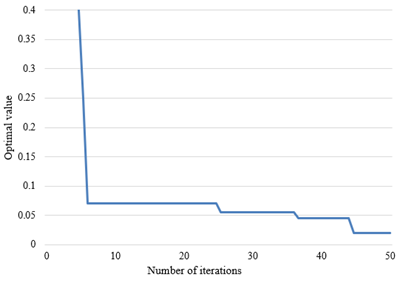Figure 5. Iterative effect of the ASFA

## 6.png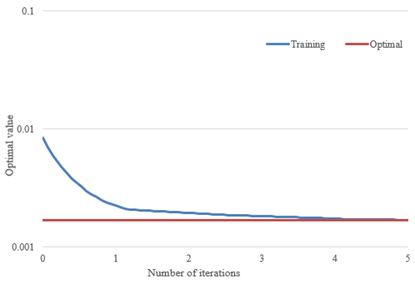Figure 6. Curve of training error

In addition, an experiment was designed to verify the effectiveness of the risk assessment model based on AFSA-optimized BPNN for smart community heating reconstruction projects. Firstly, the various parameters of the model were initialized, including learning rate, target training error, maximum number of iterations, incentive functions, learning function, training function, etc. The AFSA can update the initial connection weights and thresholds of the neural network. The termination condition of the AFSA is that the error falls below the target error, or the number of iterations reaches the maximum. Figure 5 shows the iterative effect of the ASFA.

As shown in Figure 5, BPNN was optimized ideally by the AFSA, which has a certain global convergence ability. Based on the AFSA-optimized BPNN, the proposed risk assessment model is highly feasible and researchable for smart community heating reconstruction. Next, 50 training samples were imported to the model for training. During the training, the model loss error was recorded as a curve, that is, the mean squared error (MSE) curve in Figure 6.

As shown in Figure 6, the AFSA-optimized BPNN basically tended to be stable after six iterations. The error fluctuated around 0.01. Hence, the optimization effectively overcomes the proneness of traditional BPNN to local optimum trap. After that, the risk grading effect of the improved BPNN was evaluated with 10 test samples. Table 3 provides the input layer data of the 10 test samples.

After that, the input layer data of the 10 test samples were imported to the AFSA-optimized BPNN for verification. The risk grading results of community heating reconstruction of the ten test samples are presented in Table 4.

As shown in Table 4, the accuracy of the risk grading on the ten test samples was as high as 90%. Only 1 item was misjudged, whose actual risk grade is good. The risk gradings of all the other items (whose actual risk grade is healthy, general, light risk, and heavy risk) were 100% correct. Therefore, the poorer the actual risk grade, the better the judgement by the proposed model. The results confirm that our model accurately judge the risk grades of community heating reconstruction in the 10 test samples, and could be applied to assess the risks of community heating reconstruction projects.

To verify the effectiveness of AFSA optimization for traditional BPNN, the original and improved models were tested on 15 samples. The risk assessment results of the original and improved models were summarized and compared in details. The judgement results (Figures 7 and 8) show that the improved model outputs more realistic risk grades than the original model. Table 5 compares the risk grades predicted by original and improved models.

As shown in Table 5, the improved model correctly identified 56.6% of the risk grades on the test samples. The only mistakes are about a sample of good risk and a sample of general risk. In this way, the judgement accuracy becomes much higher than that (1/2) of traditional BPNN. In practice, this paper provides a reference for the risk grading of smart community heating reconstruction in China through FCE and AFSA optimization. With the help of the model, investors and experts can evaluate the risk state of the specific community heating reconstruction project, and reasonably allocate their focus and funds to the project.

Table 3. Input layer data of the 10 test samples

 Sample number Input node 1 Input node 2 Input node 3 Input node 4 Input node 5 Input node 6 Input node 7 1 0.03898 -0.18664 0.58914 -0.25736 -0.68431 -0.37539 -4.64214 2 -0.76125 -0.5198 -1.69432 1.02410 0.77862 -2.13605 -0.57325 3 -0.06291 -0.29814 0.21982 5.78624 -0.57983 0.175311 0.27863 4 -1.5364 0.96575 4.67537 -0.26411 0.65851 -0.93542 0.5786 5 0.03668 -1.10223 0.2438 -0.27864 -0.58312 0.78365 -0.53462 6 -0.12389 -1.30215 0.18251 -0.47645 -0.53709 -0.53438 -0.67354 7 -1.23965 -0.45984 -1.53263 -0.37982 0.37946 3.46416 -0.68612 8 1.03521 -0.63846 0.17156 -0.56843 -0.44135 -0.23485 0.37205 9 1.53213 0.35317 0.54229 0.05971 0.27641 -0.47342 -0.34786 10 1.64824 1.68153 0.09234 0.03789 -0.15973 1.49651 0.67513

Table 4. Risk grading results of community heating reconstruction

 Sample number Actual risk type Output risk type Actual risk grade Judgement accuracy 1 1 1 Heavy risk 100% 95% 2 2 2 Heavy risk 100% 3 5 5 Light risk 100% 4 6 6 General 100% 5 4 4 Good 75% 6 3 5 7 2 4 8 4 3 Healthy 100% 9 2 2 10 3 3

Table 5. Risk grades predicted by original and improved models

 Risk state Actual number Wrong judgement number Accuracy Traditional BPNN Improved BPNN Traditional BPNN Improved BPNN Healthy 4 0 0 70% 100% Good 5 2 1 0% 100% General 2 1 1 100% 66% Light risk 1 1 0 0% 100% Heavy risk 3 3 0 0% 100% Total 15 7 2 60% 90%

## 7.png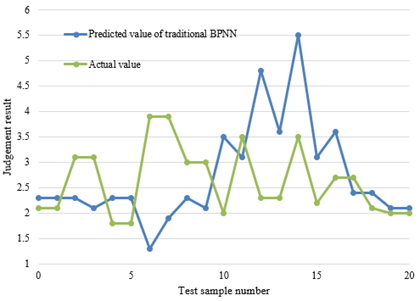Figure 7. Risk grades estimated by traditional BPNN and the actual situation

## 8.png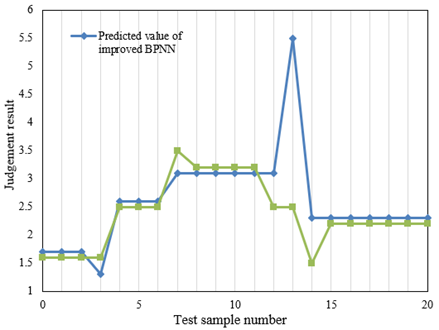Figure 8. Risk grading of the improved model vs. the actual risks

7. Conclusions

Based on ANN, this paper mainly assesses the risks of smart community heating systems. Specifically, a primary model and a secondary model were constructed for the risk assessment system of smart community heating reconstruction, the evaluation reliability was analyzed for project investment risks. Based on AFSA-optimized BPNN, the authors established a risk assessment model for smart community heating construction projects. Through experiments, the authors clarified the internal correlations between the estimated intervals of the risk assessment indices for smart community heating reconstruction, and plotted the iterative effect of the AFSA and the training error variation of the model. The training and test results on AFSA-optimized BPNN confirmed the feasibility and effectiveness of our model.

References

 Volkova, M.M., Chigvintseva, I.R. (2020). Optimization of the heat exchange system of the catalytic reforming process based on the «Tasks of Appointments». In 2020 International Multi-Conference on Industrial Engineering and Modern Technologies (FarEastCon), pp. 1-4. https://doi.org/10.1109/FarEastCon50210.2020.9271554

 Obara, S.Y., Tanno, I., Kito, S., Hoshi, A., Sasaki, S. (2008). Exergy analysis of the woody biomass Stirling engine and PEM-FC combined system with exhaust heat reforming. International Journal of Hydrogen Energy, 33(9): 2289-2299. https://doi.org/10.1016/j.ijhydene.2008.02.035

 Benaicha, F., Bencherif, K., Vivalda, J.C., Sorine, M. (2007). Water and heat conservation modelling for a reformate supplied fuel cell system. In 2007 International Conference on Power Engineering, Energy and Electrical Drives, pp. 371-376. https://doi.org/10.1109/POWERENG.2007.4380190

 Patel, K.S., Sunol, A.K. (2006). Dynamic behaviour of methane heat exchange reformer for residential fuel cell power generation system. Journal of Power Sources, 161(1): 503-512. https://doi.org/10.1016/j.jpowsour.2006.03.061

 Shudo, T., Shima, Y., Fujii, T. (2009). Production of dimethyl ether and hydrogen by methanol reforming for an HCCI engine system with waste heat recovery–Continuous control of fuel ignitability and utilization of exhaust gas heat. International Journal of Hydrogen Energy, 34(18): 7638-7647. https://doi.org/10.1016/j.ijhydene.2009.06.077

 Takeda, T. (2004). Heat transfer characteristics of the steam reformer in the HTTR hydrogen production system. In International Conference on Nuclear Engineering, 46881: 503-506. https://doi.org/10.1115/ICONE12-49415

 Yan, R., Wang, J., Cheng, Y., Ma, C., Yu, T. (2020). Thermodynamic analysis of fuel cell combined cooling heating and power system integrated with solar reforming of natural gas. Solar Energy, 206: 396-412. https://doi.org/10.1016/j.solener.2020.05.085

 Chaiyat, N., Kiatsiriroat, T. (2015). Analysis of combined cooling heating and power generation from organic Rankine cycle and absorption system. Energy, 91: 363-370. https://doi.org/10.1016/j.energy.2015.08.057

 Wang, P., Sipilä, K. (2016). Energy-consumption and economic analysis of group and building substation systems—A case study of the reformation of the district heating system in China. Renewable Energy, 87: 139-1147. https://doi.org/10.1016/j.renene.2015.08.070

 Gaber, C., Demuth, M., Schluckner, C., Hochenauer, C. (2019). Thermochemical analysis and experimental investigation of a recuperative waste heat recovery system for the tri-reforming of light oil. Energy Conversion and Management, 195: 02-312. https://doi.org/10.1016/j.enconman.2019.04.086

 Wiranarongkorn, K., Arpornwichanop, A. (2019). Assessment of heat-to-power ratio in a bio-oil sorption enhanced steam reforming and solid oxide fuel cell system. Energy Conversion and Management, 184: 8-59. https://doi.org/10.1016/j.enconman.2019.01.023

 Wu, M.L., Chen, Y.F., Li, Q., Du, S.F., Dong, Q.X., Wang, D. (2016, May). Frequency reformation of ground source heat pump system based on proportional control with grey prediction. In 2016 Chinese Control and Decision Conference (CCDC), pp. 281-6285. https://doi.org/10.1109/CCDC.2016.7532128

 Huang, C., Pan, Y., Wang, Y., Su, G., Chen, J. (2016). An efficient hybrid system using a thermionic generator to harvest waste heat from a reforming molten carbonate fuel cell. Energy Conversion and Management, 121: 186-193. https://doi.org/10.1016/j.enconman.2016.05.028

 Stutz, M.J., Grass, R.N., Loher, S., Stark, W.J., Poulikakos, D. (2008). Fast and exergy efficient start-up of micro-solid oxide fuel cell systems by using the reformer or the post-combustor for start-up heating. Journal of Power Sources, 182(2): 558-564. https://doi.org/10.1016/j.jpowsour.2008.04.020

 Kawasaki, S., Wijaya, W.Y., Watanabe, H., Okazaki, K. (2011). Waste heat recovery system through methanol steam reforming with absorption heat pump. J. Jpn. Inst. Energy, 90: 1152-1158.

 Wijaya, W.Y., Kawasaki, S., Watanabe, H., Okazaki, K. (2011). Evaluation of combined absorption heat pump–methanol steam reforming system: feasibility criterion as a measure of system performance. Energy Conversion and Management, 52(4): 1974-1982. https://doi.org/10.1016/j.enconman.2010.11.013

 Hoseinzade, L., Adams, T.A. (2017). Modeling and simulation of an integrated steam reforming and nuclear heat system. International Journal of Hydrogen Energy, 42(39): 25048-25062. https://doi.org/10.1016/j.ijhydene.2017.08.031

 Shin, G., Yun, J., Yu, S. (2017). Thermal design of methane steam reformer with low-temperature non-reactive heat source for high efficiency engine-hybrid stationary fuel cell system. International Journal of Hydrogen Energy, 42(21): 14697-14707. https://doi.org/10.1016/j.ijhydene.2017.04.053

 Kushi, T., Ohashi, M., Kato, R., Ito, T., Inagaki, S., Amaha, S. (2017). Evaluation of Performance and Heat Balance of Dry Reforming SOFC System. ECS Transactions, 78(1): 2409. https://doi.org/10.1149/07801.2409ecst

 Kim, J.H., Kwon, O.C. (2011). A micro reforming system integrated with a heat-recirculating micro-combustor to produce hydrogen from ammonia. International Journal of Hydrogen Energy, 36(3): 1974-1983.

 Kawasaki, S., Yanto Wijaya, W., Watanabe, H., Okazaki, K. (2011). A Proposal of Waste Heat Recovery System Through Methanol Steam Reforming Integrated with Absorption Heat Pump. In ASME/JSME Thermal Engineering Joint Conference, 38921: T20098. https://doi.org/10.1115/AJTEC2011-44253

 Liso, V., Olesen, A.C., Nielsen, M.P., Kær, S.K. (2011). Performance comparison between partial oxidation and methane steam reforming processes for solid oxide fuel cell (SOFC) micro combined heat and power (CHP) system. Energy, 36(7): 4216-4226. https://doi.org/10.1016/j.energy.2011.04.022

 Wu, W., Pai, C.C. (2009). Control of a heat-integrated proton exchange membrane fuel cell system with methanol reforming. Journal of Power Sources, 194(2): 920-930. https://doi.org/10.1016/j.jpowsour.2009.05.030

 Shudo, T., Shima, Y., Fujii, T. (2009). Production of dimethyl ether and hydrogen by methanol reforming for an HCCI engine system with waste heat recovery–continuous control of fuel ignitability and utilization of exhaust gas heat. International Journal of Hydrogen Energy, 34(18): 7638-7647. https://doi.org/10.1016/j.ijhydene.2009.06.077

 Purnima, P., Jayanti, S. (2017). Water neutrality and waste heat management in ethanol reformer-HTPEMFC integrated system for on-board hydrogen generation. Applied Energy, 199: 169-179. https://doi.org/10.1016/j.apenergy.2017.04.069

 Wiranarongkorn, K., Im-Orb, K., Ponpesh, P., Patcharavorachot, Y., Arpornwichanop, A. (2017). Design and evaluation of the sorption enhanced steam reforming and solid oxide fuel cell integrated system with anode exhaust gas recirculation for combined heat and power generation. Chemical Engineering Transactions, 57: 97-102. https://doi.org/10.3303/CET1757017

 Shin, G., Yun, J., Yu, S. (2017). Thermal design of methane steam reformer with low-temperature non-reactive heat source for high efficiency engine-hybrid stationary fuel cell system. International Journal of Hydrogen Energy, 42(21): 14697-14707. https://doi.org/10.1016/j.ijhydene.2017.04.053